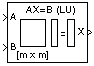# LU Solver

Solve AX = B when A is a square matrix

• Library:
• DSP System Toolbox / Math Functions / Matrices and Linear Algebra / Linear System Solvers

•## Description

The LU Solver block solves the linear system AX = B by applying LU factorization, where:

• A is an M-by-M square matrix input through the A port.

• B is an M-by-N matrix input through the B port.

• X is the M-by-N output matrix and is the unique solution to the equations.

## Ports

### Input

expand all

Specify the input M-by-M square matrix A through this port. Inputs A and B must have the same number of rows.

Data Types: `single` | `double`
Complex Number Support: Yes

Specify the B matrix in the AX = B equation as an M-by-N matrix or an M-by-1 vector.

When you specify an M-by-1 vector, the block treats the length-M unoriented vector input at port B as an M-by-1 matrix. Inputs A and B must have the same number of rows.

Data Types: `single` | `double`
Complex Number Support: Yes

### Output

expand all

The block returns the output as an M-by-N matrix or a M-by-1 matrix. The size of X matrix is same as the size of the B matrix.

Data Types: `single` | `double`
Complex Number Support: Yes

## Block Characteristics

 Data Types `double` | `single` Direct Feedthrough `no` Multidimensional Signals `no` Variable-Size Signals `no` Zero-Crossing Detection `no`

## Algorithms

The LU algorithm factors a row-permuted variant (Ap) of the square input matrix A as

`${A}_{p}=LU$`

where L is a lower triangular square matrix with unity diagonal elements, and U is an upper triangular square matrix.

The matrix factors are substituted for Ap in

`${A}_{p}X={B}_{p}$`

where Bp is the row-permuted variant of B, and the resulting equation

`$LUX={B}_{p}$`

is solved for X by substituting Y = UX, and solving two triangular systems.

`$\begin{array}{l}LY={B}_{p}\hfill \\ UX=Y\hfill \end{array}$`

## Version History

Introduced before R2006a